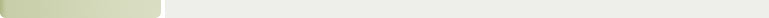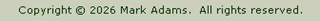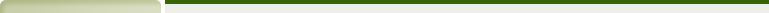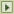FeedbackMembers Forum"Casio FX Programs"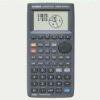Casio fx-7400G PLUS Casio fx-9860GII (SD)

These programs MUST NOT be sold - collectively or individually, electronically as software or pre-installed on graphic calculators without the express permission of the author.

These programs may be distributed freely but must maintain their copyright status.

They are supplied "as-is" and the author accepts no responsibility for any errors caused by their use.

Casio Power Graphic fx-7400G PLUS

my_progs_fxi  (7Kb)  Latest update:  17th January 2014

A zipped Casio FXI file containing all of the 32 programs listed below.  The file can only be opened and transferred to a Calculator using the Casio FX software and an interface cable.

Line to Plane  (253Kb)  ** NEW JANUARY 2014

This is a new program for the fx-7400G.  It calculates the 3D co-ordinates of a point at the intersection of a line (defined by 2 points) to a plane (defined by 3 points).  Useful if trying to co-ordinate a hidden point (where it is not possible to measure a distance).

A program listing and explanation is also included

Casio Programs v4.11  (677Kb)

A pdf document illustrating how the programs work along with some examples.  Thanks Cliff for spotting the typos.

Program Listings  (214 Kb)

Listings of the programs in zipped PDF format.

 Program Description 1 1PT~CFIX Calculates centre of circle from 2 HA's & 1 Distance 2 2PT~CFIX Circle centre from 2 points and Radius 3 3D~CFIX Circle centre from three 3D points 4 3D~DIST Calculates 3D distance between 2 points 5 3PT~CFIX Circle centre from three 2D points 6 ANGLE Calculates Angle between two lines defined by 4 points 7 AREA Calculates Area given co-ordinates of Polygon corners 8 BRG~DIST Calculates Bearing and Distance between 2 points 9 CIRCLE Calculates Circle Edge given one ordinate 10 COLUMN Finds column centre from 2 corners and a dimension 11 CURVE Calculates setting-out points on a curve at a given interval 12 CUT~AREA Land sub-division program 13 HAVERSIN Determines Starting/Ending WCB between 2 global points 14 INT~BEAR Intersection by Bearings (2 points and 2 bearings) 15 INT~DIST Intersection by Distances (2 points and 2 distances) 16 INT~LINE Intersection of two lines (defined by 4 points) 17 LEVELS Simple Backsight/Foresight reduction program 18 LN2PLANE Calculates the Intersection point of a Line to a Plane 19 MEAN~XYZ Averages two co-ordinates 20 NOTEBOOK Stores up to 9 variables 21 OFFSET Determines the Positive/Negative Offset and Chainage of a point 22 POLYGON Calculates the Bearing & Distances of points around a Polygon 23 PT2LINE Calculates the 3D distance of a Point to a Line and IP co-ords 24 PT2PLANE Calculates the 3D distance of a Point from a Plane 25 RADIAL Calculates the Bearing & Distances of points from a single point 26 RESECT Resection by Angles to three known points 27 SURVEY Calculates co-ordinates from Angles and Distances 28 SURVEY2D Calculates 3D co-ordinates from HA, VA & Slope/Hz Distances 29 TRANSFRM Transforms one grid to another given 2 known points 30 TRAVERSE Calculates provisional co-ords by angles and forward distances 31 UNI~COLS Full list of Universal Column sizes 32 VECTORS Extension of vectors from two given points 33 VERTICAL Determines verticality by measuring HA over a known distance

Casio fx-9860GII (SD)

fx-9860_g1m  (16Kb)

The fx-7400G PLUS (.fxi) files have been converted to .G1M format.  Unzip and install as required.

** Updated version of Levelling program for fx-9860GII

 Program Description 1 1PT~CFIX Calculates centre of circle from 2 HA's & 1 Distance 2 2PT~CFIX Circle centre from 2 points and Radius 3 3D~CFIX Circle centre from three 3D points 4 3D~DIST Calculates 3D distance between 2 points 5 3PT~CFIX Circle centre from three 2D points 6 ANGLE Calculates Angle between two lines defined by 4 points 7 AREA Calculates Area given co-ordinates of Polygon corners 8 BRG~DIST Calculates Bearing and Distance between 2 points 9 CIRCLE Calculates Circle Edge given one ordinate 10 COLUMN Finds column centre from 2 corners and a dimension 11 CURVE Calculates setting-out points on a curve at a given interval 12 CUT~AREA Land sub-division program 13 HAVERSIN Determines Starting/Ending WCB between 2 global points 14 INT~BEAR Intersection by Bearings (2 points and 2 bearings) 15 INT~DIST Intersection by Distances (2 points and 2 distances) 16 INT~LINE Intersection of two lines (defined by 4 points) 17 LEVELING Intersight reductions & Full level run (no adjustment) ** 18 LN2PLANE Calculates the Intersection point of a Line to a Plane 19 MEAN~XYZ Averages two co-ordinates 20 NOTEBOOK Stores up to 9 variables 21 OFFSET Determines the Positive/Negative Offset and Chainage of a point 22 POLYGON Calculates the Bearing & Distances of points around a Polygon 23 PT2LINE Calculates the 3D distance of a Point to a Line and IP co-ords 24 PT2PLANE Calculates the 3D distance of a Point from a Plane 25 RADIAL Calculates the Bearing & Distances of points from a single point 26 RESECT Resection by Angles to three known points 27 SURVEY Calculates co-ordinates from Angles and Distances 28 SURVEY2D Calculates 3D co-ordinates from HA, VA & Slope/Hz Distances 29 TRANSFRM Transforms one grid to another given 2 known points 30 TRAVERSE Calculates provisional co-ords by angles and forward distances 31 UNI~COLS Full list of Universal Column sizes 32 VECTORS Extension of vectors from two given points 33 VERTICAL Determines verticality by measuring HA over a known distance

See fx-7400-G PLUS for illustrated examples and program listings.